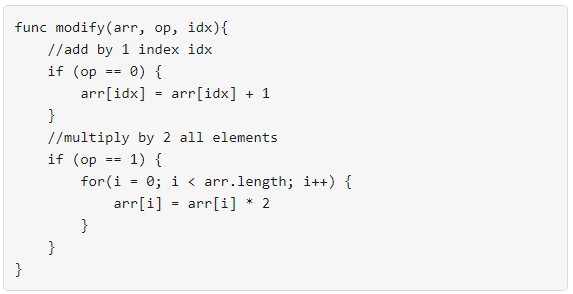# 得到目标数组的最少函数调用次数

## 1558. 得到目标数组的最少函数调用次数 (Medium)```输入：nums = [1,5]

```

```输入：nums = [2,2]

```

```输入：nums = [4,2,5]

```

```输入：nums = [3,2,2,4]

```

```输入：nums = [2,4,8,16]

```

• `1 <= nums.length <= 10^5`
• `0 <= nums[i] <= 10^9`

[贪心算法]